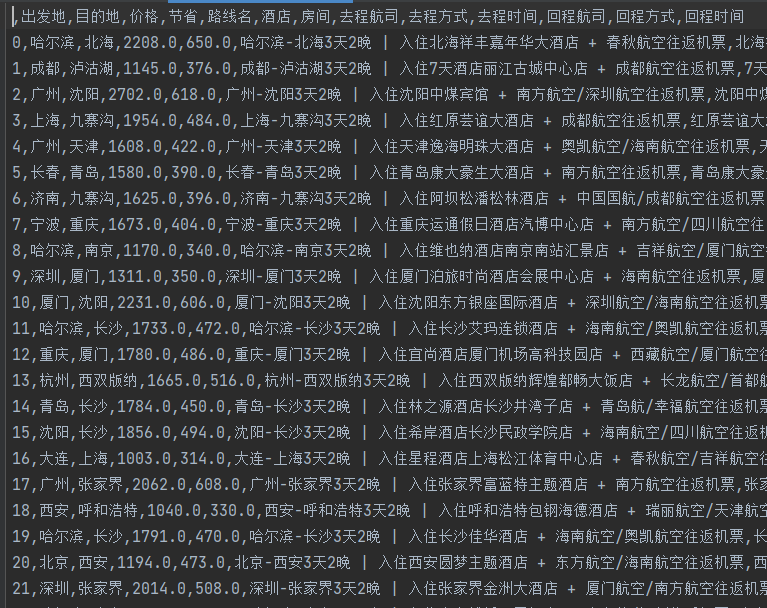# 入门Python数据清洗

## Demo#### 笔记

``````- 数据清洗常用包
- import pandas as pd
- import numpy as np

- 导入数据集
- .....
- 示例代码：

- 查看数据结构
- 查看数据形状
- df.shape()
- 查看数据结构(查看数据行、是否有空值、数据类型等)
- df.info()
- 查看数据描述性统计信息(数值型数据的描述统计)
- df.describe()

- 简单数据处理
- 列名
- df.columns
- 列名去除空格
- 导入列名
- col = df.columns.values
- 列表推导执行(strip的作用是去除前后空格)
- [x.strip() for x in col]
- 示例代码
- df.columns = [x.strip() for x in col]
- df.columns

- 数据求值（平均值、中位数、最大值、最小值、求和、标准差）
- 平均值和指定列平均值
- df.mean()
- df["价格"].mean()
- 中位值和指定列平均值
- df.median()
- 同上
- 最大值和最小值
- df['价格'].max()
- df['价格'].min()
- 求和和标准差
- df['价格'].sum()
- df['价格'].std()
- 整体查看
- df.describe()

- 重复值处理
- df.duplicated()
- 返回布尔类型值，确定缺失值位置
- 仅支持全部特征值相同时才返回True
- 支持从前向后、从后向前的查找模式
- df[df.duplicated()]
- 查找被判断为重复值的记录
- df.duplicated().sum()
- 被判断为重复值数量
- df.drop_duplicates()
- 删除重复值（没有修改原数据，想当初创建出新的数据集）
- df.drop_duplicates(inplace=True)
- 删除重复值(在原数据上操作)
- df.index = range(df.shape)
- 重置索引

- 异常值处理
- 价格
- df.describe().T
- 查看数据值
- sta = (df['价格'] - df['价格'].mean()) / df['价格'].std()
- df[sta.abs() > 3]
- 三倍标准差衡量是否异常
- 节省（节省价格高于或等于价格属于异常）
- sum(df.价格 > df.节省)
- 计算正常值数量
- df[df.价格 < df.节省]
- 找出异常值
- 异常值合并后取除索引
- delindes = pd.co ncat([df[df.价格 < df.节省], df[sta.abs() > 3]]).index
- 依据索引删除异常值
- df.drop(delindes, inplace=True)

- 缺失值处理
- df.notnull()
- 查看不是缺失值的数据（布尔类型）
- df.isnull()
- 查看缺失值（布尔类型）
- df.isnull().sum()
- 查看每一列的缺失数据个数
- 缺失值填补（出发地）
- 确实的出发地可以在路线名中找到补值
- df.loc[df.出发地.isnull(), "出发地"] = [str(x)[:2] for x in df.loc[df.出发地.isnull(), '路线名']]
- 缺失值填补（目的地)
- 同上
- df.loc[df.目的地.isnull(), '目的地'] = str(df.loc[df.目的地.isnull(), "路线名"].values)[5:7]
- 缺失值填补（价格)
- df['价格'].fillna(round(df['价格'].mean(), 0), inplace=True)
- 缺失值填补（节省)
- df['节省'].fillna(round(df['节省'].mean(), 0), inplace=True)

- 文本型数据处理
- 正则表达式提取``````

#### 笔记文件

THE END